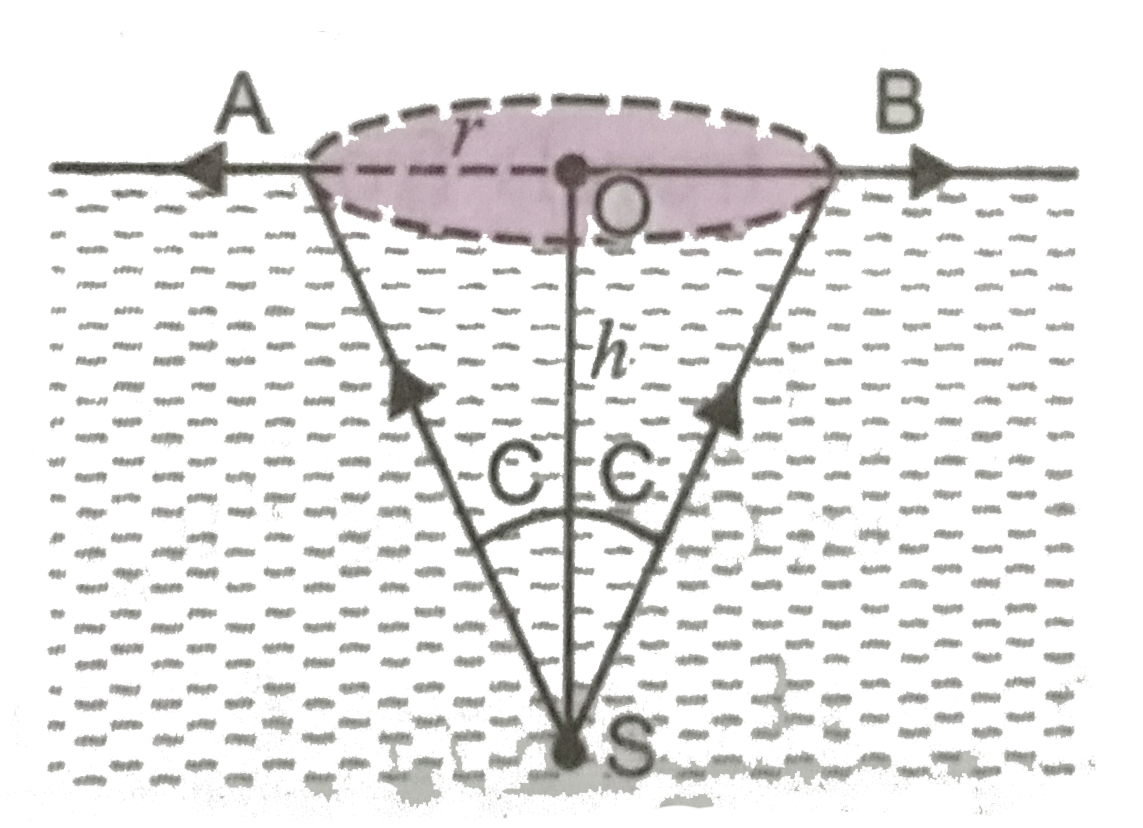# A point source of light S is placed at the bottom of a vessel containing a liquid of refractive index 5//3. A person is viewing the source from ab

30 views
in Physics
closed
A point source of light S is placed at the bottom of a vessel containing a liquid of refractive index 5//3. A person is viewing the source from above the surface. There is an opaque disc of radius 1 cm floating on the surface. The centre of disc lies vertically above the source O. The liquid from the vessel is gradually drained out through a tap. What is the maximum height of the liquid for which the source cannot be seen at all.

by (117k points)
selected

In Fig., let OS = h be the max. height of liquid above the source for which the source cannot be seen at all.:. angle OSA = C
Any other ray from S will be totally internally refracted because in that case, angle of incidence will be greater them the critical angle.
In DeltaOSA, sin C = (OA)/(SA) = (r )/(sqrt(r^(2) + h^(2))) = (1)/(mu)
or (1)/(mu_(2)) = (r )/(r^(2) + h^(2))
r^(2) + h^(2) = mu^(2) r^(2)
h^(2) = r^(2)(mu^(2) - 1)
h = r sqrt(mu^(2) - 1) = 1 sqrt((5//3)^(2) - 1
h = (4)/(3) = 1.33 cm